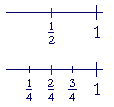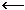S k i l l
i n
A R I T H M E T I C

Lesson 22

EQUIVALENT FRACTIONS

 1. What are equivalent fractions? Equivalent fractions have different names but the same value; they are at the same place on the number line; they have the same relationship to 1.

Here is an elementary example:

 12 = 24 .12 is 1 of 2 equal parts of 1. 24 is 2 of 4 equal parts.  Each

fraction is one half of 1.

As fractions of a unit of measure, equivalent fractions are equal measurements.

 12 inch = 24 inch.

 2. How can we know when two fractions are equivalent?Both the numerator and denominator of one fraction have been multiplied by the same number, and those products are the numerator and denominator of the equivalent fraction.
 23 and 1015 are equivalent fractions.

Every fraction has a ratio to 1. Equivlent fractions will have the same ratio to 1.

Each numerator and denominator will have all the properties of ratios, specifically the theorem of the same multiple.

In the lesson on comparing fractions, however, see Problem 2.

 Example 1.   Name three fractions that are equivalent to 56 .

 1012 1518 5060

To create them, we multiplied both 5 and 6 by the same number.  First by 2, then by 3, then by 10.

 3. How do we convert a fraction to an equivalent fraction with a larger denominator?Multiply the original denominator so that it equals the larger denominator. Multiply the numerator by the same number.
 34 = 6 × 36 × 4 = 1824 .

(Compare Lesson 20, Problem 2c.)

Example 2.    Write the missing numerator:

 67 = ? 28

Answer.   To make 7 into 28, we have to multiply it by 4.  Therefore, we must also multiply 6 by 4:

 67 = 244 × 6284 × 7

In practice, to find the multiplier, mentally divide the original denominator into the new denominator, and then multiply the numerator by that quotient.  That is, say:

"7 goes into 28 four times. Four times 6 is 24."

Compare Lesson 18, Example 5.  Every property of ratios applies to fractions.

Example 3.   Write the missing numerator:

 58 = ? 48

Answer.   "8 goes into 48 six times.  Six times 5 is 30."

 58 = 3048 .

In actual problems, we convert two (or more) fractions so that they have equal denominators.  When we do that, it is easy to compare them (see the next Lesson, Question 3), and equal denominators are necessary in order to add or subtract them (Lesson 25).  For we can only add or subtract quantities that have the same name, that is, that are units of the same kind; and it is the denominator of a fraction that names the unit. (Lesson 21.)

Now, since 15, for example, is a multiple of 5, we say that 5 is a divisor of 15.  (5 is not a divisor of 14, because 14 is not a multiple of 5.)

5 is also a divisor of 20.  5 is a common divisor of 15 and 20.

(15 and 14 have no common divisors, except 1, which is a divisor of every number.)

4.   What denominator should we choose
when the denominators of two fractions have no common divisors,
 23 and 58
and we want to convert them to equivalent fractions with equal denominators?

Choose the product of the denominators.

 Example 4.   Convert 23 and 58 to equivalent fractions with

equal denominators.

Answer.   The denominators 3 and 8 have no common divisors (except 1).  Therefore, as a common denominator, choose 24.

 23 = 1624 . 58 = 1524 .
 To convert 23 , we said, "3 goes into 24 eight times. Eight

times 2 is 16."

 To convert 58 , we said, "8 goes into 24 three times. Three

times 5 is 15."

Once we convert to a common denominator, we could then know

 that 23 is greater than 58 .  Because when fractions have

equal denominators, then the larger the numerator, the larger the fraction. (Lesson 20, Question 11.)

Also, we could now add those fractions:

 23 + 58 = 1624 + 1524 = 3124 .

We can choose the product of denominators even when the denominators have a common divisor. But their product will not then be their lowest common multiple (Lesson 23). The student should prefer the lowest common multiple because smaller numbers make for simpler calculations.

Same ratio

When fractions are equivalent, their numerators and denominators are in the same ratio.  That in fact is the best definition of equivalent fractions.

 12 = 24 .

1 is half of 2.  2 is half of 4.  In fact, any fraction where the

 numerator is half of the denominator will be equivalent to 12 .
 12 = 24 = 36 = 5 10 = 8 16 .

1 is half of 2.  2 is half of 4.  3 is half of 6.  5 is half of 10.  And so on.  These are all at the same place on the number line.Example 5. 4 12 and 5 15 are equivalent because each

numerator is a third of its denominator.

Example 6.   Write the missing numerator:

 7 28 = ? 16

Answer.  7 is a quarter of 28.  And a quarter of 16 is 4.

 7 28 = 4  16 .

7 is to 28  as  4 is to 16.

 7 28 and 4 16 are equivalent.

How to simplify, or reduce, a fraction

The numerator and denominator of a fraction are called its terms.  To simplify or reduce a fraction means to make the terms smaller.  To accomplish that, we divide both terms by a common divisor.

 Example 7. 2436 = 24 ÷ 436 ÷ 4 = 69 = 6 ÷ 39 ÷ 3 = 23 .
 2436 , 69 , and 23 are equivalent fractions.
 Of those three 23 has the lowest terms -- we cannot

divide any further.

We like to express a fraction with its lowest terms because it gives a better sense of its value, and it makes for simpler calculations.

 5. How do we reduce a fraction to its lowest terms? Keep dividing both terms by a common divisor. Or, take the same part of both terms.

 Example 8.   Reduce to lowest terms: 15 21 .

Answer.  15 and 21 have a common divisor, 3.

 1521 = 5"3 goes into 15 five times."7"3 goes into 21 seven times."

Or, take a third of both 15 and 21.

 Example 9.   Reduce 200 1200 .

Answer.  When the terms have the same number of 0's, we may ignore them.

 2001200 = 16

Effectively, we have divided 200 and 1200 by 100. (Lesson 2, Question 10.)

 Example 10.   Write as a mixed number: 20 8 .

Solution.  Divide 20 by 8.  "8 goes into 20 two (2) times (16) with 4 left over."

 20 8 =  2 48 =  2 12 .
 48 is equivalent to 12 .

Or, we could reduce first.  20 and 8 have a common divisor 4:

 20  8 = 5"4 goes into 20 five times."2"4 goes into 8 two times." = 2½.

Notice that we are free to interpret the same symbol

 20 8
 in various ways.  It is the fraction 20 8 .  It is 20 divided by 8.

And it indicates "the ratio of 20 to 8."

 Example 11.   Reduce 55 .

Any fraction in which the numerator and denominator are equal, is equal to 1.

 SummaryAt this point, please "turn" the page and do some Problems.

or

Continue on to the next Section.